JEE  >  Test: Comprehension Based Questions: Matrices and Determinants

# Test: Comprehension Based Questions: Matrices and Determinants

Test Description

## 13 Questions MCQ Test Maths 35 Years JEE Main & Advanced Past year Papers | Test: Comprehension Based Questions: Matrices and Determinants

Test: Comprehension Based Questions: Matrices and Determinants for JEE 2023 is part of Maths 35 Years JEE Main & Advanced Past year Papers preparation. The Test: Comprehension Based Questions: Matrices and Determinants questions and answers have been prepared according to the JEE exam syllabus.The Test: Comprehension Based Questions: Matrices and Determinants MCQs are made for JEE 2023 Exam. Find important definitions, questions, notes, meanings, examples, exercises, MCQs and online tests for Test: Comprehension Based Questions: Matrices and Determinants below.
Solutions of Test: Comprehension Based Questions: Matrices and Determinants questions in English are available as part of our Maths 35 Years JEE Main & Advanced Past year Papers for JEE & Test: Comprehension Based Questions: Matrices and Determinants solutions in Hindi for Maths 35 Years JEE Main & Advanced Past year Papers course. Download more important topics, notes, lectures and mock test series for JEE Exam by signing up for free. Attempt Test: Comprehension Based Questions: Matrices and Determinants | 13 questions in 20 minutes | Mock test for JEE preparation | Free important questions MCQ to study Maths 35 Years JEE Main & Advanced Past year Papers for JEE Exam | Download free PDF with solutions
 1 Crore+ students have signed up on EduRev. Have you?
Test: Comprehension Based Questions: Matrices and Determinants - Question 1

### A square matrix in which all the elements except at least the one element in the diagonal are zeros is said to be a

Detailed Solution for Test: Comprehension Based Questions: Matrices and Determinants - Question 1

A diagonal matrix is a matrix in which all of the elements not on the diagonal of a square matrix are 0.

Test: Comprehension Based Questions: Matrices and Determinants - Question 2

### PASSAGE - 1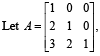and U1, U2 and U3 are columns of a 3 × 3 matrix U. If column matrices U1, U2 and U3 satisfying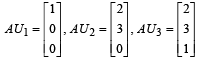evaluate as directed in the following questions Q. The sum of the elements of the matrix U–1 is

Detailed Solution for Test: Comprehension Based Questions: Matrices and Determinants - Question 2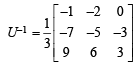⇒ Sum of elements of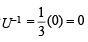Test: Comprehension Based Questions: Matrices and Determinants - Question 3

### PASSAGE - 1and U1, U2 and U3 are columns of a 3 × 3 matrix U. If column matrices U1, U2 and U3 satisfyingevaluate as directed in the following questions Q. The value of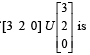Detailed Solution for Test: Comprehension Based Questions: Matrices and Determinants - Question 3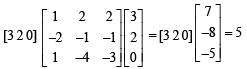Test: Comprehension Based Questions: Matrices and Determinants - Question 4

PASSAGE - 2

Let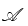be the set of all 3× 3 symmetric matrices all of whose entries are either 0 or 1. Five of these entries are 1 and four of them are 0.

Q. The number of matrices inis

Detailed Solution for Test: Comprehension Based Questions: Matrices and Determinants - Question 4

Each element of set A is 3 × 3 symmetric matrix with five of its entries as 1 and four of its entries as 0, we can keep in diagonal either 2 zero and one 1 or no zero and three 1 so that the left over zeros and one’s are even in number.
Hence taking 2 zeros and one 1 in diagonal the possible cases are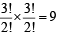and taking 3 ones in diagonal the possible cases are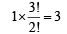∴ Total elements A can have = 9 + 3 = 12

Test: Comprehension Based Questions: Matrices and Determinants - Question 5

PASSAGE - 2

Letbe the set of all 3× 3 symmetric matrices all of whose entries are either 0 or 1. Five of these entries are 1 and four of them are 0.

Q. The number of matrices A infor which the system of linear equations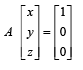Detailed Solution for Test: Comprehension Based Questions: Matrices and Determinants - Question 5

The given system will have unique solution if | A |≠ 0 which is so for the matrices.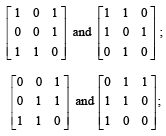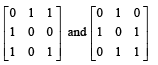which are 6 in number.

Test: Comprehension Based Questions: Matrices and Determinants - Question 6

PASSAGE - 2

Letbe the set of all 3× 3 symmetric matrices all of whose entries are either 0 or 1. Five of these entries are 1 and four of them are 0.

Q. The number of matrices A infor which the system of linear equations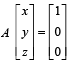Detailed Solution for Test: Comprehension Based Questions: Matrices and Determinants - Question 6

For the given system to be inconsistent |A| = 0. The matrices for which | A | = 0 are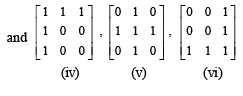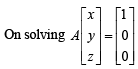We find for A = (i)
By Cramer’s rule D1 = 0 = D2=D3
∴ infinite many solution
For A  = (ii)
By Cramer ’s rule D1 ≠ 0
⇒ no solution i.e. inconsistent.
Similarly we find the system as inconsistent in cases (iii), (v) and (vi).
Hence for four cases system is inconsistent.

Test: Comprehension Based Questions: Matrices and Determinants - Question 7

PASSAGE - 3

Let p be an odd prime number and Tp be the following set of 2 × 2 matrices :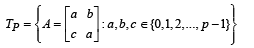Q. The number of A in Tp such that A is either symmetric or skew-symmetric or both, and det(A) divisible by p is

Detailed Solution for Test: Comprehension Based Questions: Matrices and Determinants - Question 7

If A is symmetrie then b = c
⇒ A = a2 – b2 = (a+b)(a –b)
Which is divisible by p if (a + b) is divisible by p or (a – b) is divisible by p.
Now (a + b) is divisible by p if (a, b) can take values (1, p – 1), (2, p – 2),(3, p – 3),...(p–1, 1)
∴ (p – 1)ways.
Also (a – b) is divisible by p only when a – b = 0 i.e. a = b, then (a, b) can take values (0,0) (1,1), (2,2)..... (pÝ, p–2)
∴ p ways.
If A is skew symmetric, then a = 0 and b = – c or b + c = 0 which gives |A| = 0 when b2
∴ b = 0, c = 0 But this possibility is already included when A is symmetric and (a, b) = (0, 0).
Again if A is both symmetric and skew symmetric, then clearly A is null matrix which case is already included.
Hence total number of ways = p + (p – 1) = 2p – 1

Test: Comprehension Based Questions: Matrices and Determinants - Question 8

PASSAGE - 3

Let p be an odd prime number and Tp be the following set of 2 × 2 matrices :Q. The number of A in Tp such that the trace of A is not divisible by p but det (A) is divisible by p is

[Note: The trace of a matrix is the sum of its diagonal entries.]

Detailed Solution for Test: Comprehension Based Questions: Matrices and Determinants - Question 8

Trace A = a + a = 2a is not divisible by p ⇒ a is not divisible by p
⇒ a ≠ 0
But |A| is divisible by p
⇒ a2 – bc is divisible by p
It will be so if on dividing a2 by p suppose we get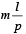then on dividing bc by p we should get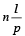for some
integeral values of m, n and l. i.e. the remainder should be same in each case, so that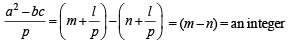For this to happen a can take any value from 1 to p–1, also if b takes any value from 1 to p–1 then c should take only that value corresponding to which the remainder is same.
∴ No. of ways = (p – 1) × (p – 1) × 1 = (p – 1)2.

Test: Comprehension Based Questions: Matrices and Determinants - Question 9

PASSAGE - 3

Let p be an odd prime number and Tp be the following set of 2 × 2 matrices :Q. The number of A in Tp such that det (A) is not divisible by p is

Detailed Solution for Test: Comprehension Based Questions: Matrices and Determinants - Question 9

Total number of matrices = total number of ways a, b, c can be selected = p × p × p = p3.
Number of ways when det (A) is divisible by p and trace (A) ≠ 0 are equal to number of ways det (A) is divisible by p and trace (A) is not divisible by p = (p – 1)2

Also number of ways when det (A) is divisible by p and trace A = 0 are the ways when bc is multiple of p
⇒ b = 0 or c = 0 for b = 0, c can take values 0, 1, 2, ......., p – 1
For c = 0, b can take values 0, 1, 2, ............, p – 1
Here (b, c) = (0, 0) is coming twice.
∴ Total ways of selecting b and c = p + p – 1 = 2p – 1
∴ Total number of ways when det (A) is divisible by p = (p – 1)2 + 2p – 1 = p2 Hence the number of ways when det (A) is not divisible by p = p3 – p2.

Test: Comprehension Based Questions: Matrices and Determinants - Question 10

PASSAGE - 4

Let a, b and c be three real numbers satisfying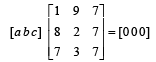...(E)

Q. If the point P(a, b, c), with reference to (E), lies on the plan e 2x + y + z = 1, then the value of 7a + b + c is

Detailed Solution for Test: Comprehension Based Questions: Matrices and Determinants - Question 10

From equation (E), we get
a + 8b + 7c = 0
9a + 2b + 3c = 0
a + b + c  = 0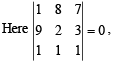Therefore system has infinite many solutions.
Solving these, we get b = 6a and c = – 7a
Now (a, b, c) lies on 2x + y + z = 1
⇒ b = 6, c = – 7
∴  2a + 6a – 7a = 1 ⇒ a = 1
∴ 7a + b + c = 7 + 6 – 7 = 6 ⇒ b = 6, c = – 7

Test: Comprehension Based Questions: Matrices and Determinants - Question 11

PASSAGE - 4

Let a, b and c be three real numbers satisfying...(E)

Q. Let ω be a solution of x3 – 1 = 0 with Im (ω) > 0 , if a = 2 with b and c satisfying (E), then the value of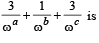equal to

Detailed Solution for Test: Comprehension Based Questions: Matrices and Determinants - Question 11

If a = 2 then b = 12, c = –14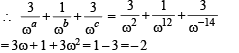Test: Comprehension Based Questions: Matrices and Determinants - Question 12

PASSAGE - 4

Let a, b and c be three real numbers satisfying...(E)

Q. Let b = 6, with a and c satisfying (E). If a and b are the roots of the quadratic equation ax2 + bx + c = 0, then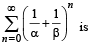Detailed Solution for Test: Comprehension Based Questions: Matrices and Determinants - Question 12

If b = 6 then a = 1, c = – 7
∴ Equation becomes x2 + 6x – 7 = 0 or (x + 7) (x – 1) = 0 whose roots are 1 and –7.
Let α = 1 and β = – 7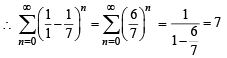Test: Comprehension Based Questions: Matrices and Determinants - Question 13

Consider the system of equations

x – 2y + 3z = –1
–x + y – 2z = k
x – 3y + 4z = 1

STATEMENT - 1 : The system of equations has no solution for k ¹ 3   and

STATEMENT-2 : The determinant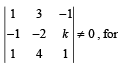k ≠ 3 .

Detailed Solution for Test: Comprehension Based Questions: Matrices and Determinants - Question 13

The given equations are
x – 2y + 3z = – 1
– x + y – 2z = k
x – 3y + 4z =1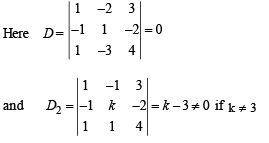∴ If k ≠ 3 , the system has no solutions.
Hence statement-1 is true and statement-2 is a correct explanation for statement - 1.

## Maths 35 Years JEE Main & Advanced Past year Papers

132 docs|70 tests
 Use Code STAYHOME200 and get INR 200 additional OFF Use Coupon Code
Information about Test: Comprehension Based Questions: Matrices and Determinants Page
In this test you can find the Exam questions for Test: Comprehension Based Questions: Matrices and Determinants solved & explained in the simplest way possible. Besides giving Questions and answers for Test: Comprehension Based Questions: Matrices and Determinants, EduRev gives you an ample number of Online tests for practice

## Maths 35 Years JEE Main & Advanced Past year Papers

132 docs|70 tests